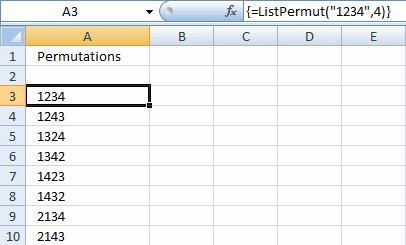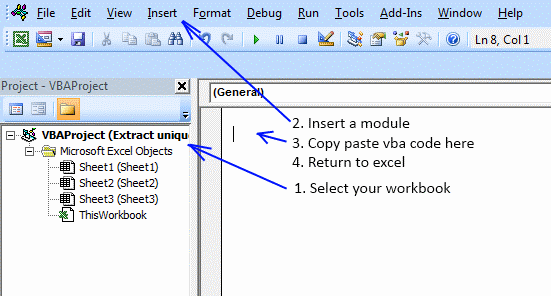Author: Oscar Cronquist Article last updated on February 19, 2019Maximum Number Allowed is 4 digit and the number is from 0 to 9.

After I fill in the number i want it to automatic permutate the numbers and list in details, example if i key in 1234 and the list will be:

1234, 1243, 1423, 4123, 1324, 1342, 1432, 4132, 3124, 3142, 3412, 4312, 2134, 2143, 2413, 4213, 2314, 2341, 2431, 4231, 3214, 3241, 3421, 4321.

This udf creates permutations from a text string. You can also choose how many letters in each permutation.

Array formula in cell A3:A26:

=ListPermut("1234",4)

How to create this array formula

1. Select cell range A3:A26
2. Type above array formula in formula bar
3. Press and hold Ctrl + Shift
4. Press Enter once
5. Release all keys

VBA code

```Function ListPermut(str As String, num As Integer)
'Permutations without repetition

Dim c, r, p As Long
Dim rng() As Long, temp As Long, i As Long
Dim temp1 As Long, y() As Long, d As Long
Dim tmpOut(), tmpArr() As Variant
Dim j As Integer
Dim a As Boolean
ReDim tmpArr(0)
ReDim tmpOut(0)

For j = 1 To Len(str)
tmpArr(UBound(tmpArr)) = Mid(str, j, 1)
ReDim Preserve tmpArr(UBound(tmpArr) + 1)
Next j

ReDim Preserve tmpArr(UBound(tmpArr) - 1)
p = WorksheetFunction.Permut(Len(str), Len(str))
ReDim rng(1 To p, 1 To Len(str))

For c = 1 To Len(str)
rng(1, c) = c
Next c

For r = 2 To p

For c = 1 To num
tmpOut(UBound(tmpOut)) = tmpOut(UBound(tmpOut)) & tmpArr(rng(r - 1, c) - 1)
Next c

If UBound(tmpOut) <> 0 Then
If tmpOut(UBound(tmpOut)) = tmpOut(UBound(tmpOut) - 1) Then
tmpOut(UBound(tmpOut)) = ""
Else
ReDim Preserve tmpOut(UBound(tmpOut) + 1)
End If
Else
ReDim Preserve tmpOut(UBound(tmpOut) + 1)
End If

For c = Len(str) To 1 Step -1
If rng(r - 1, c - 1) < rng(r - 1, c) Then temp = c - 1 Exit For End If Next c For c = Len(str) To 1 Step -1 rng(r, c) = rng(r - 1, c) Next c For c = Len(str) To 1 Step -1 If rng(r - 1, c) > rng(r - 1, temp) Then
temp1 = rng(r - 1, temp)
rng(r, temp) = rng(r - 1, c)
rng(r, c) = temp1
ReDim y(Len(str) - temp)
i = 0
For d = temp + 1 To Len(str)
y(i) = rng(r, d)
i = i + 1
Next d
i = 0
For d = Len(str) To temp + 1 Step -1
rng(r, d) = y(i)
i = i + 1
Next d
Exit For
End If
Next c

If r = p Then
For c = 1 To num
tmpOut(UBound(tmpOut)) = tmpOut(UBound(tmpOut)) & tmpArr(rng(r, c) - 1)
Next c
If tmpOut(UBound(tmpOut)) = tmpOut(UBound(tmpOut) - 1) Then
ReDim Preserve tmpOut(UBound(tmpOut) - 1)
End If
End If

Next r

ListPermut = Application.Transpose(tmpOut)

End Function
```

Where to copy vba code?

Press Alt+F11### Get the Excel fileChris_ListPermut_norep.xls